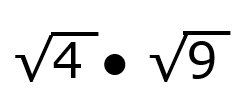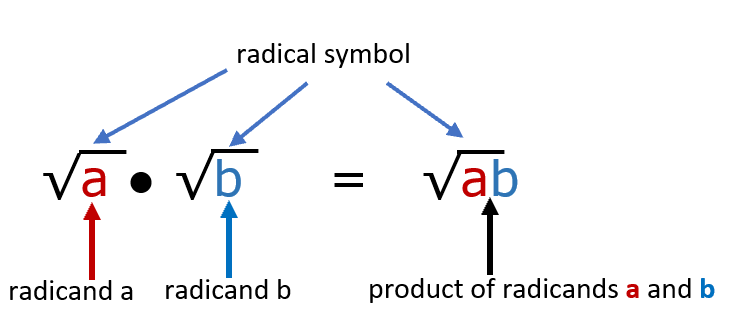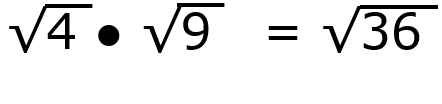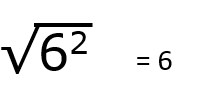Home > Math Topics > Algebra >

#### High School - HSA-REI.A.2

This is often a section where students will not necessarily be confused but lead on the wrong path. When ever you work with radicals, after a break from them, we highly encourage teachers to revisit the basic concepts of a radical. Remind them that even though the symbol looks like those we have seen in units on division, this symbol (the radical) tells us to calculate the root of the value. By itself the symbol signifies that we are looking for the square root specifically. A square root of a value is a number that if we were to multiply it be itself, it gives use that value. In this section we will be working with perfect and not so perfect squares. In addition, we will include negative roots at times too. We encourage you to review all those basic calculations before helping them approach finding the products of these values. You must understand where the accelerator and brake are before you can drive a car.

### Printable Worksheets And Lessons

• Step-by-Step Lesson- You will see that we always provided this fulol work through of a problem at the bottom of this page.
• Guided Lesson - Three problems for you to get some practice in on.
• Guided Lesson Explanation - Each of the three problems above are full spelled out for you in detail.
• Independent Practice - We cover all the basic, intermediate, and advanced skills all on one sheet.
• Matching Worksheet - While this may be an easier exercise, I find it really helps students learn trends that they overlook by just doing the basic operations.#### Homework Sheets

There is a nice flow of difficulty here. You will work towards more advanced techniques with each successive problem.

• Homework 1 - We get you started with just two terms. Pay attention to the negative symbols and the values that spin out of them.
• Homework 2 - We add an additional term and the instace of parentheses bracket that you will need to manage along the way.
• Homework 3 - Solve each side of the brackets first before you begin to compile everything.

#### Practice Worksheets

It is time to see how well we understand this topic.

• Practice 1 - If you made your way through all the homework, this sheet should be quick.
• Practice 2 - Remember to concentrate on where the terms are and how they affect one another.
• Practice 3 - Reverse engineer your solution to check your answers. If you can do that, the quizzes below will be a breeze for you.

#### Math Skill Quizzes

The quizzes follow the same scheme that can be seen with the homework and practice sheets.

• Quiz 1 - If you have a good deal of difficulty with this one, make sure to review more before proceeding further.
• Quiz 2 - The location of the bracket does not matter.
• Quiz 3 - To make these problems quicker to understand we used smaller values, so that the calculator does not get in the way.

### How About An Example Exercise?

Find the product of the radical values.There are basic rules that apply to finding the products of radicals. It can be all summed up with this rule flow:The word radicand is the math term that resides with the square root symbol. This flow shows how when multiplying two radicals, we combine the two radicands to simplify this operation. We will now apply this our original problem.The product of these radicands is a perfect square of 36. 36 = 6 x 6 = 62

We can now apply this to simplify our perfect square.The radical and exponent cancel one another out.

### Where Do I Use This in Real Life?

Radical expressions are commonly used in applications of geometry and trigonometry. Now do not run off and say, "Math class? I knew it!" We all use these applications and never even know it. Did you know the paper that you write on is a direct √2 ratio of the longer side to the shorter side? Because radicals are heavily used in circle geometry, just about everything that is engineered on the planet in the shape of a circle must brush up with it at some point. That would include wheels, tires, and yes, your favorite household cookie. Speaking of construction, did you know that most housing and apartment structure are valued by square footage. So, if you wanted to get an idea of how long the sides of the house or a specific room were, you would take the square root of the square footage. That can be applied to anything that involves square footage.